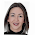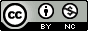## Monday, July 25, 2011

### Scatterplot matrices in R

I just discovered a handy function in R to produce a scatterplot matrix of selected variables in a dataset. The base graphics function is pairs(). Producing these plots can be helpful in exploring your data, especially using the second method below.

Try it out on the built in iris dataset. (data set gives the measurements in cm of the variables sepal length and width and petal length and width, respectively, for 50 flowers from each of 3 species of iris. The species are Iris setosa, versicolor, and virginica).

```# Load the iris dataset.
data(iris)

# Plot #1: Basic scatterplot matrix of the four measurements
pairs(~Sepal.Length+Sepal.Width+Petal.Length+Petal.Width, data=iris)```
Looking at the pairs help page I found that there's another built-in function, panel.smooth(), that can be used to plot a loess curve for each plot in a scatterplot matrix. Pass this function to the lower.panel argument of the pairs function. The panel.cor() function below can compute the absolute correlation between pairs of variables, and display these in the upper panels, with the font size proportional to the absolute value of the correlation.

```# panel.smooth function is built in.
# panel.cor puts correlation in upper panels, size proportional to correlation
panel.cor <- function(x, y, digits=2, prefix="", cex.cor, ...)
{
usr <- par("usr"); on.exit(par(usr))
par(usr = c(0, 1, 0, 1))
r <- abs(cor(x, y))
txt <- format(c(r, 0.123456789), digits=digits)
txt <- paste(prefix, txt, sep="")
if(missing(cex.cor)) cex.cor <- 0.8/strwidth(txt)
text(0.5, 0.5, txt, cex = cex.cor * r)
}

# Plot #2: same as above, but add loess smoother in lower and correlation in upper
pairs(~Sepal.Length+Sepal.Width+Petal.Length+Petal.Width, data=iris,
lower.panel=panel.smooth, upper.panel=panel.cor,
pch=20, main="Iris Scatterplot Matrix")```

Finally, you can produce a similar plot using ggplot2, with the diagonal showing the kernel density.

```# Plot #3: similar plot using ggplot2
# install.packages("ggplot2") ## uncomment to install ggplot2
library(ggplot2)
plotmatrix(with(iris, data.frame(Sepal.Length, Sepal.Width, Petal.Length, Petal.Width)))```

See more on the pairs function here.

...

Update:  A tip of the hat to Hadley Wickham (@hadleywickham) for pointing out two packages useful for scatterplot matrices. The gpairs package has some useful functionality for showing the relationship between both continuous and categorical variables in a dataset, and the GGally package extends ggplot2 for plot matrices.

1.There's also a function in the psych package (built on top of the two lattice functions you describe above) which has scatterplots in the upper triangle, loess fits on the lower and histograms on the diagonal.

2.That would be the highly recommended pairs.panels() function. It does also paint ellipses. Have a look at these examples:
http://www.oga-lab.net/RGM2/func.php?rd_id=psych:pairs.panels

3.I appreciate the link to the psych package function. pairs.panels() adds some nice functionality!

4.Also, check out Zach Meyer's post on graphically analyzing variable interactions, which shows even more advanced scatterplot matrix plots.

5.Very useful, thank you.

You can get rid of the measures in the axis by using xaxt = 'n' and yaxt = 'n' inside the pairs function

6.Very useful function!

An improvement would be:

r <- cor(x, y) # remove abs()
text(0.5, 0.5, txt, cex = cex.cor * abs(r)) # and move to this line

In this way, we plot the real (positive or negative) value of the correlation coefficient and not only positive values. So, we also can draw higher sizes in strong negative correlation coefficients.

Note: Only a member of this blog may post a comment.